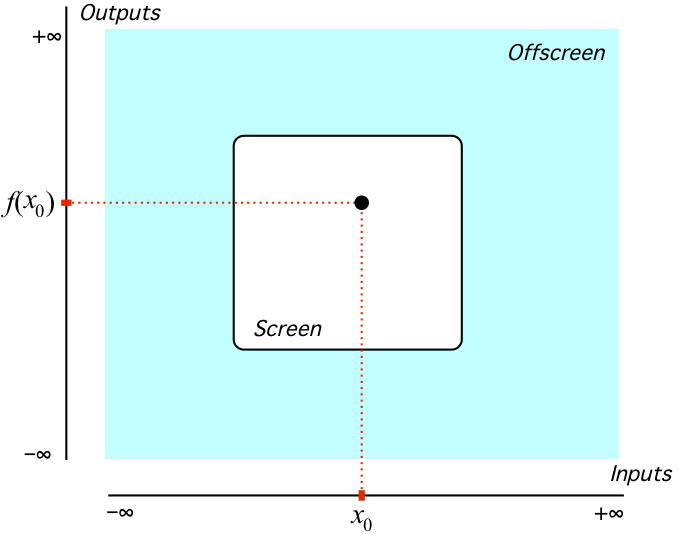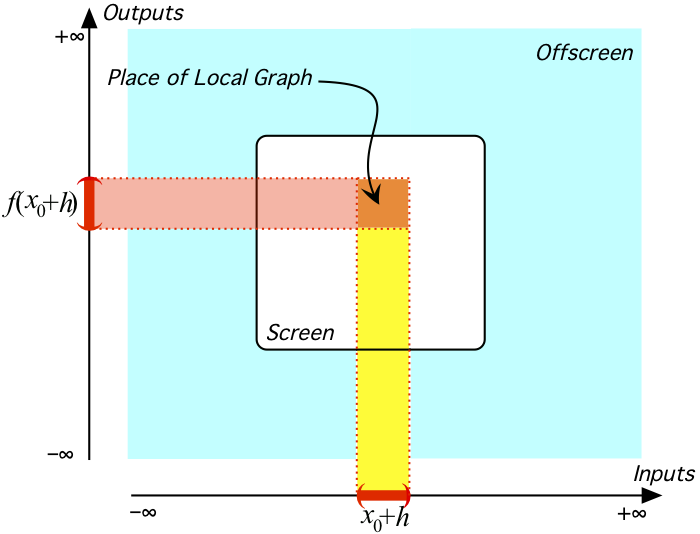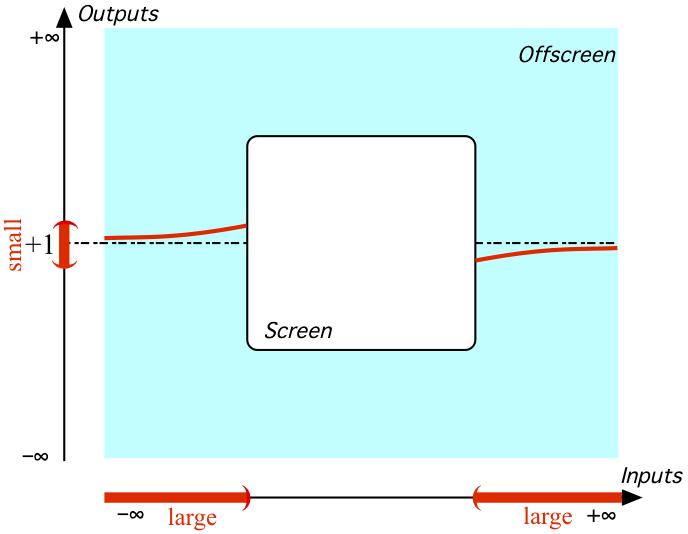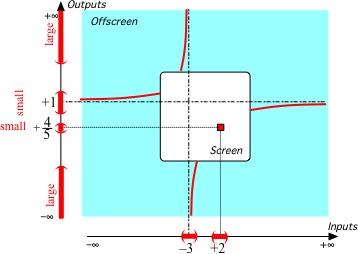# Laurent Polynomial Approximations?1

Laurent polynomials are nice because, like decimal numbers in arithmetic, they allow us to approximate and therefore to get what we want at the least possible cost2. But how does one sell such an idea in a culture which still prefers miles, feet and inches to kilometers, meters and centimeters and, even more to the point, fractions to decimals? Being an obdurate curmudgeon, though, I will try to make a case for "approximate algebra" in a series of pieces.

I will begin by showing here how Laurent polynomial approximations provide a nice alternative to the highly questionable but unfortunately usual recipe for graphing a function: "Pick a few inputs, compute the outputs, and join the plot points smoothly." (If nothing else, how about the fact that there could be any number of poles inbetween the plot points not to mention oscillations? Moreover, how can a few plot points foster any understanding of the function's behavior?)

The general idea will be to "thicken the plot", that is, given an input, instead of going for the plot point at the given inputwhich registers only the output at the given input, we will want the local graph near the given input.

because, in addition to registering with its height the outputs for inputs near the given input, a local graph also registers with its slope and its concavity how the outputs change near the given input.

So, instead of just computing outputs at given inputs, we will compute outputs near given inputs. But then the immediate question is: near which given inputs? The answer, though, is rather reasonable: look at what's $$large$$. More precisely, $$large$$ inputs but also $$bounded$$ inputs whose nearby inputs have $$large$$ outputs. (In other words, we will want the local graphs near $$∞$$ and near the poles, if any.)

For instance, in the case of the function $$x \xrightarrow{\hspace{5mm}f\hspace{5mm}}f(x)=\frac{x^{2}-4}{x^{2}+x-6}$$:
1. We declare that $$x$$ is near $$\infty$$: \begin{align*} x\text{ near } \infty \xrightarrow{\hspace{5mm}f\hspace{5mm}}f(x)&=\frac{x^{2}-4}{x^{2}+x-6} \end{align*} We approximate separately Numerator $$f(x)$$ and Denominator $$f(x)$$ keeping in mind that $$x$$ is $$large$$: \begin{align*} \hspace{37mm}&=\frac{x^{2} + [...]}{x^{2} + [...]} \end{align*} We divide the monomials (short division): \begin{align*} \hspace{37mm}&= +1 + [...] \end{align*} where $$[...]$$, read as "something too small to matter here", is a proto Bachmann-Landau little $$o$$.

However, since here we don't want just the height (sometimes, though, that's all we need as when we just want the sign of the ouput near $$∞$$), but also the slope and the concavity, what we ignored above was not "too small to matter" and we need the long division which we stop as soon as we get to a term with slope and concavity: \begin{align*}x\text{ near } \infty \xrightarrow{\hspace{5mm}f\hspace{5mm}}f(x)&=\frac{x^{2}-4}{x^{2}+x-6} \\ &= +1 -x^{-1} + [...] \end{align*} which gives us the local graph of $$f$$ near $$∞$$2. The question now is whether we may just join the local graph near $$∞$$ smoothly across the screen as inor if there might not be $$bounded$$ inputs $$x_{0}$$ near which $$f$$ returns $$large$$ outputs (AKA poles). So, we compute the outputs near $$x_{0}$$, namely a generic bounded input: \begin{align*} x\gets x_{0}+h \xrightarrow{\hspace{2mm}f\hspace{2mm}}f(x_{0}+h) = \frac{\text{Numerator }f(x_{0}+h)}{\text{Denominator } f(x_{0}+h)}&=\frac{(x_{0}+h)^{2}-4}{(x_{0}+h)^{2}+(x_{0}+h)-6} \\ &=\frac{[x_{0}^{2}-4]+[2x_{0}]h+[+1]h^{2}}{[x_{0}^{2}+x_{0}-6]+[2x_{0}+1]h+[+1]h^{2}} \end{align*} Now for $$f(x_{0}+h)$$ to be $$large$$, either

• $$\text{Numerator }f(x_{0}+h)$$ would have to be $$large$$. But since $$[x_{0}^{2}-4]$$ is $$bounded$$ and $$[2x_{0}]h+[+1]h^{2}$$ is $$small$$, $$\text{Numerator }f(x_{0}+h)$$ cannot be $$large$$. (Of course, $$\text{Numerator }f$$ being a polynomial function, $$\text{Numerator }f(x)$$ can be $$large$$ only near $$\infty$$.)
• or
• $$\text{Denominator }f(x_{0}+h)$$ would have to be $$small$$. So, $$[x_{0}^{2}+x_{0}-6]$$, the only term that is not $$small$$, would have to be $$0$$. We compute $$\text{Discriminant } [x^{2}+x-6]=+25$$ which gives us that the constant term $$[x_{0}^{2}+x_{0}-6]$$ will be $$0$$ when $$x_{0}$$ is $$+2$$ or $$-3$$ and $$\text{Denominator }f(x_{0}+h)$$ will be $$small$$ near $$+2$$ and $$-3$$.

So, $$f(x_{0}+h)$$ may be $$large$$ only near $$+2$$ and/or $$-3$$. To find out, we look at the outputs near $$+2$$ and near $$-3$$:

• We declare that $$x$$ is near $$+2$$: \hspace{-54mm} \begin{align*}x\gets+2+h \xrightarrow{\hspace{5mm}f\hspace{5mm}}f(+2+h)=\frac{\text{Numerator }f(+2+h)}{\text{Denominator } f(+2+h)}&=\frac{(+2+h)^{2}-4}{(+2+h)^{2}+(+2+h)-6} \\ &=\frac{+[+4]h +[+1]h^{2}}{+[+5]h +[+1]h^{2}} \end{align*} We approximate separately $$\text{Numerator }f(+2+h)$$ and $$\text{Denominator }f(+2+h)$$ keeping in mind that $$h$$ is $$small$$: \begin{align*} \hspace{30mm}&=\frac{+4h + [...]}{+5h + [...]} \end{align*} We divide (short division): \begin{align*} \hspace{30mm}&= +\frac{4}{5} + [...] \end{align*} So, $$+2$$ is not a pole (and, by the way and the same token, nor is $$+2$$ a zero) and we need not bother with the local graph near $$+2$$.

• We declare that $$x$$ is near $$-3$$: \hspace{-54mm} \begin{align*}x\gets-3+h \xrightarrow{\hspace{5mm}f\hspace{5mm}}f(-3+h)\frac{\text{Numerator }f(-3+h)}{\text{Denominator } f(-3+h)}&=\frac{(-3+h)^{2}-4}{(-3+h)^{2}+(-3+h)-6} \\ &=\frac{[+5]+[-6]h +[+1]h^{2}}{+[-5]h +[+1]h^{2}} \end{align*} We approximate separately $$\text{Numerator }f(-3+h)$$ and $$\text{Denominator }f(-3+h)$$ keeping in mind that $$h$$ is $$small$$: \begin{align*} \hspace{30mm}&=\frac{+5 + [...]}{-5h + [...]} \end{align*} We divide (short division): \begin{align*} \hspace{30mm}&= -h^{-1} + [...] \end{align*} So, $$-3$$ is a pole and we get the local graph near $$-3$$.

We thus have the offscreen graphand I will leave the essential bounded graph, where by "essential" I mean forced by the offscreen graph, to the reader's imagination.

3. The essential bounded graph gives us some rather useful information about $$f$$ namely that $$f$$ has no essential extremum, $$f$$ has an essential zero between $$-3$$ and $$+2$$, $$f$$ is essentially piecewise increasing, and $$f$$ has an essential concavity sign-change---whose existence we already knew from just the local graph near $$∞$$, whose location is: $$x_{\text{concavity sign-change}}=-3$$.

4. However, this essential information is only about what would be visible from far away and the essential bounded graph says nothing about non-essential features of $$f$$ (i.e. deformable to the vanishing point) such as oscillations and/or waverings. To detect non-essential features, we start again from: \begin{align*}x\gets x_{0}+h \xrightarrow{\hspace{5mm}f\hspace{5mm}}f(x_{0}+h)&=\frac{(x_{0}+h)^{2}-4}{(x_{0}+h)^{2}+(x_{0}+h)-6} \\ &= \frac{[x_{0}^{2}-4]+[2x_{0}]h+[+1]h^{2}}{[x_{0}^{2}+x_{0}-6]+[2x_{0}+1]h+[+1]h^{2}} \end{align*} but to get the Laurent Polynomial Approximation near $$x_{0}$$ we need the long division (in ascending powers of $$h$$) which usually is a somewhat formidable affair. However, the constant term \begin{align*} \hspace{26mm}&= \frac{[x_{0}^{2}-4]+[...]}{[x_{0}^{2}+x_{0}-6]+[...]} \\ &=\frac{x_{0}^{2}-4}{x_{0}^{2}+x_{0}-6} +[...] \end{align*} already shows that $$f$$ is continuous and differentiability requires only the linear term.

We all have our tricks. Laurent polynomial approximations, though, are not a trick and there would be no point in your trying anything like the above in your Monday morning Precalculus class because it would have no more chance to mean anything to your students in the long run than were you to decide in a Developmental Arithmetic course to devote an hour to decimal approximations. We are talking about a mindset so, in both cases, you have to reconstruct the whole course content.
Of course, as physicist David Hestenes of Geometric Algebra fame said at the outset of his 2002 Oersted lecture:
Course content is taken [by many] as given, so the research problem is how to teach it most effectively. This approach [...] has produced valuable insights and useful results. However, it ignores the possibility of improving pedagogy by reconstructing course content. (Emphasis added.)
But, as Kipling would have said, that is another story---which I will try to tell in subsequent pieces.
2. Why Laurent polynomials? If only because $8\,765.432 = \left.8x^{+3} + 7x^{+2} + 6x^{+1} + 5x^{0} + 4x^{-1} + 3x^{-2} + 2x^{-3} \right|_{x\gets 10}$